Launch this interactive notebook in Binder ⇒## Detector¶

### ThresholdAD¶

ThresholdAD compares each time series value with given thresholds.

In the following example, we detect time points when temperature is above 30C or below 15C.

In :
import pandas as pd
s = pd.read_csv('./data/temperature.csv', index_col="Time", parse_dates=True, squeeze=True)
from adtk.data import validate_series
s = validate_series(s)

In :
from adtk.detector import ThresholdAD
threshold_ad = ThresholdAD(high=30, low=15)
anomalies = threshold_ad.detect(s)

In :
from adtk.visualization import plot
plot(s, anomaly=anomalies, ts_linewidth=1, ts_markersize=3, anomaly_markersize=5, anomaly_color='red', anomaly_tag="marker");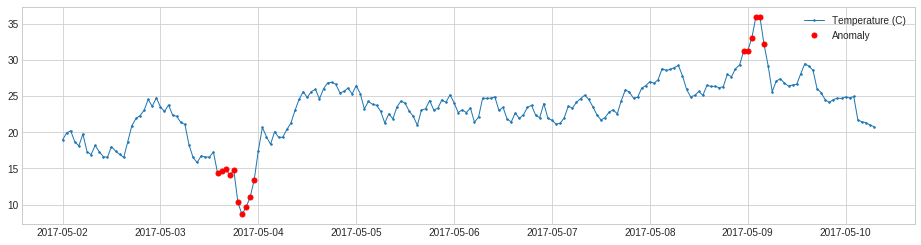### QuantileAD¶

QuantileAD compares each time series value with historical quantiles.

In the following example, we detect time points when temperature is above 99% percentile or below 1% percentile.

In :
from adtk.detector import QuantileAD
quantile_ad = QuantileAD(high=0.99, low=0.01)
anomalies = quantile_ad.fit_detect(s)

In :
plot(s, anomaly=anomalies, ts_linewidth=1, ts_markersize=3, anomaly_markersize=5, anomaly_color='red', anomaly_tag="marker");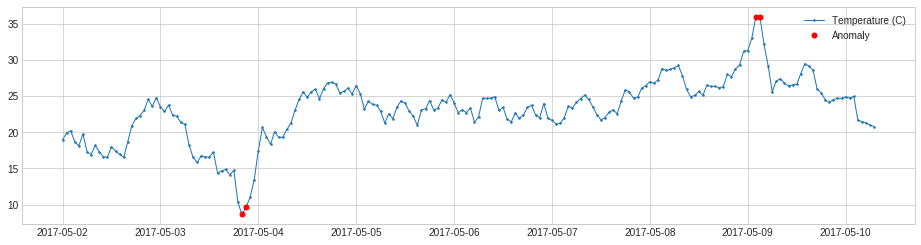### InterQuartileRangeAD¶

InterQuartileRangeAD is another widely used detector based on simple historical statistics is based on interquartile range (IQR). When a value is out of the range defined by $[Q_1 - c \times IQR,\ Q_3 + c \times IQR]$ where $IQR = Q_3 - Q_1$ is the difference between 25% and 75% quantiles. This detector is usually preferred to QuantileAD in the case where only a tiny portion or even none of training data is anomalous.

In :
from adtk.detector import InterQuartileRangeAD
iqr_ad = InterQuartileRangeAD(c=1.5)
anomalies = iqr_ad.fit_detect(s)

In :
plot(s, anomaly=anomalies, ts_linewidth=1, ts_markersize=3, anomaly_markersize=5, anomaly_color='red', anomaly_tag="marker");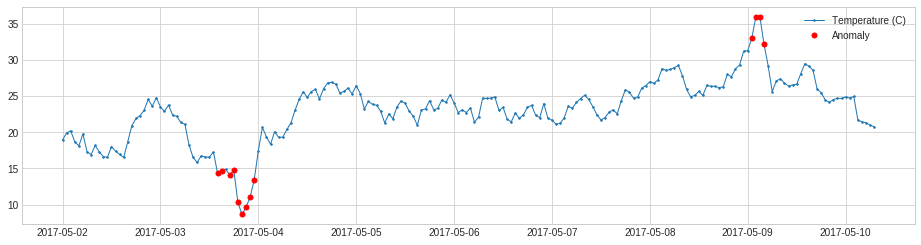### GeneralizedESDTestAD¶

GeneralizedESDTestAD detects anomaly based on generalized extreme Studentized deviate (ESD) test.

Please note a key assumption of generalized ESD test is that normal values follow an approximately normal distribution. Please only use this detector when this assumption holds.

In :
from adtk.detector import GeneralizedESDTestAD
esd_ad = GeneralizedESDTestAD(alpha=0.3)
anomalies = esd_ad.fit_detect(s)

In :
plot(s, anomaly=anomalies, ts_linewidth=1, ts_markersize=3, anomaly_markersize=5, anomaly_color='red', anomaly_tag="marker");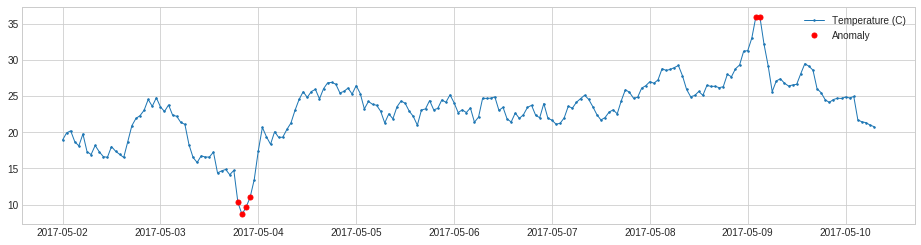### PersistAD¶

PersistAD compares each time series value with its previous values. Internally, it is implemented as a pipenet with transformer DoubleRollingAggregate.

In the following example, we detect anomalous positive changes of price.

In :
s = pd.read_csv('./data/price_short.csv', index_col="Time", parse_dates=True, squeeze=True)
s = validate_series(s)

In :
from adtk.detector import PersistAD
persist_ad = PersistAD(c=3.0, side='positive')
anomalies = persist_ad.fit_detect(s)
plot(s, anomaly=anomalies, ts_linewidth=1, ts_markersize=3, anomaly_color='red');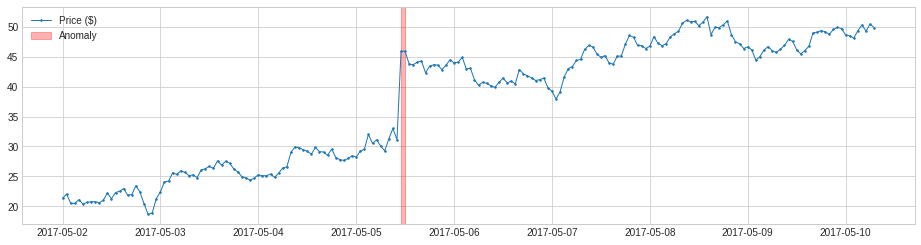By default, PersistAD only check one previous value, which is good at capturing additive anomaly in short-term scale, but not in long-term scale because it is too near-sighted.

In the following example, it fails to capture meaningful drops of price in a longer time scale.

In :
s = pd.read_csv('./data/price_long.csv', index_col="Time", parse_dates=True, squeeze=True)
s = validate_series(s)

In :
persist_ad = PersistAD(c=1.5, side='negative')
anomalies = persist_ad.fit_detect(s)
plot(s, anomaly=anomalies, anomaly_color='red');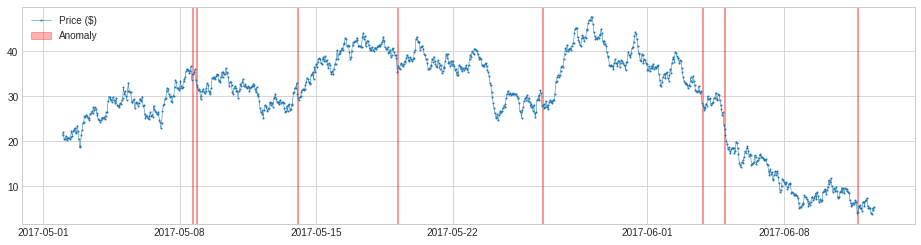We may change the parameter window to a number greater than 1, and the detector will compare a value to the median or mean of its preceding time window. This will capture anomalous changes in mid- to long-term scale, because it is less near-sighted. In the same example as above, it detects drops of price in the long-term scale successfully.

In :
persist_ad.window = 24
anomalies = persist_ad.fit_detect(s)

In :
plot(s, anomaly=anomalies, anomaly_color='red');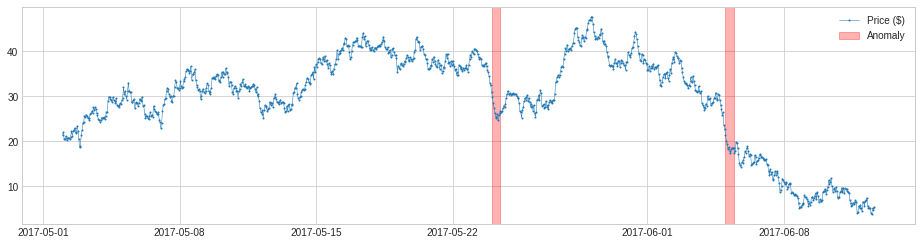### LevelShiftAD¶

LevelShiftAD detects shift of value level by tracking the difference between median values at two sliding time windows next to each other. It is not sensitive to instantaneous spikes and could be a good choice if noisy outliers happen frequently. Internally, it is implemented as a pipenet with transformer DoubleRollingAggregate.

In the following example, we detect shift point of CPU usage.

In :
s = pd.read_csv('./data/cpu.csv', index_col="Time", parse_dates=True, squeeze=True)
s = validate_series(s)

In :
from adtk.detector import LevelShiftAD
level_shift_ad = LevelShiftAD(c=6.0, side='both', window=5)
anomalies = level_shift_ad.fit_detect(s)
plot(s, anomaly=anomalies, anomaly_color='red');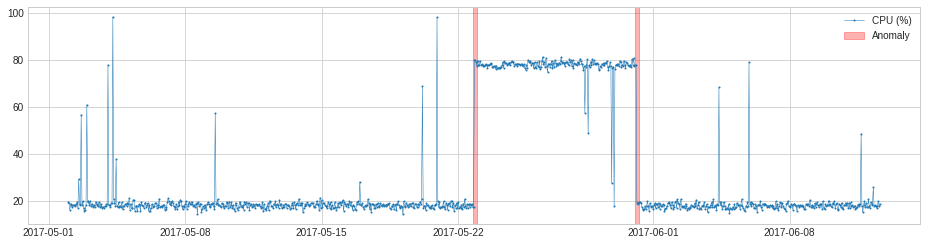### VolatilityShiftAD¶

VolatilityShiftAD detects shift of volatility level by tracking the difference between standard deviations at two sliding time windows next to each other. Internally, it is implemented as a pipenet with transformer DoubleRollingAggregate.

In the following example, we detect positive shift of volatility of seismic amplitude which indicates start of earthquake.

In :
s = pd.read_csv('./data/seismic.csv', index_col="Time", parse_dates=True, squeeze=True)
s = validate_series(s)

In :
from adtk.detector import VolatilityShiftAD
volatility_shift_ad = VolatilityShiftAD(c=6.0, side='positive', window=30)
anomalies = volatility_shift_ad.fit_detect(s)
plot(s, anomaly=anomalies, anomaly_color='red');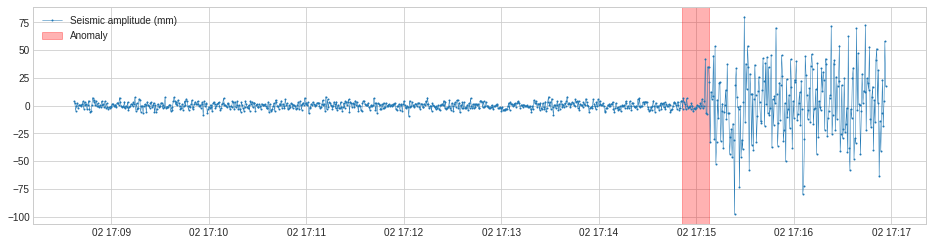### SeasonalAD¶

SeasonalAD detects anomalous violations of seasonal pattern. Internally, it is implemented as a pipenet with transformer ClassicSeasonalDecomposition.

In the following example, we detect unusual traffic, which mostly happened on major holidays.

In :
s = pd.read_csv('./data/seasonal.csv', index_col="Time", parse_dates=True, squeeze=True)
s = validate_series(s)

In :
from adtk.detector import SeasonalAD
seasonal_ad = SeasonalAD(c=3.0, side="both")
anomalies = seasonal_ad.fit_detect(s)
plot(s, anomaly=anomalies, ts_markersize=1, anomaly_color='red', anomaly_tag="marker", anomaly_markersize=2);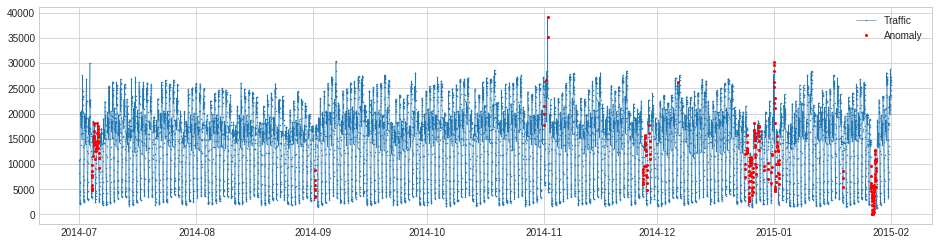### AutoregressionAD¶

AutoregressionAD detects anomalous changes of autoregressive behavior in time series. Internally, it is implemented as a pipenet with transformers Retrospect and RegressionResidual.

For the same example as above, we detect violation of usual regressive behavior in traffic history.

In :
s = pd.read_csv('./data/seasonal.csv', index_col="Time", parse_dates=True, squeeze=True)
s = validate_series(s)

In :
from adtk.detector import AutoregressionAD
autoregression_ad = AutoregressionAD(n_steps=7*2, step_size=24, c=3.0)
anomalies = autoregression_ad.fit_detect(s)
plot(s, anomaly=anomalies, ts_markersize=1, anomaly_color='red', anomaly_tag="marker", anomaly_markersize=2);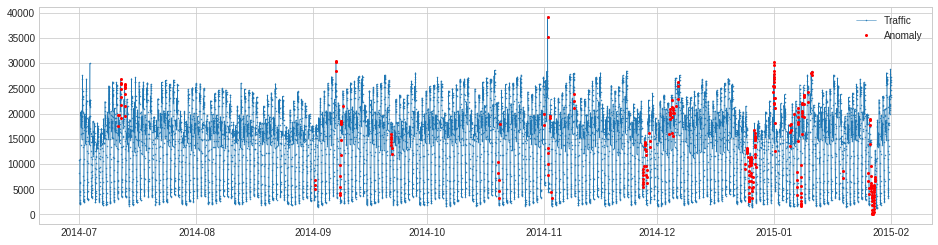### MinClusterDetector¶

MinClusterDetector treats multivariate time series as independent points in a high-dimensional space, divides them into clusters, and identifies values in the smallest cluster as anomalous. This may help capturing outliers in high-dimensional space.

In the following example, we detect anomalous changes of relationship between the speed of a generator and power generated. The violation of regular relationship indicates equipment failure.

In :
df = pd.read_csv('./data/generator.csv', index_col="Time", parse_dates=True, squeeze=True)
df = validate_series(df)

In :
from adtk.detector import MinClusterDetector
from sklearn.cluster import KMeans
min_cluster_detector = MinClusterDetector(KMeans(n_clusters=3))
anomalies = min_cluster_detector.fit_detect(df)
plot(df, anomaly=anomalies, ts_linewidth=1, ts_markersize=3, anomaly_color='red', anomaly_alpha=0.3, curve_group='all');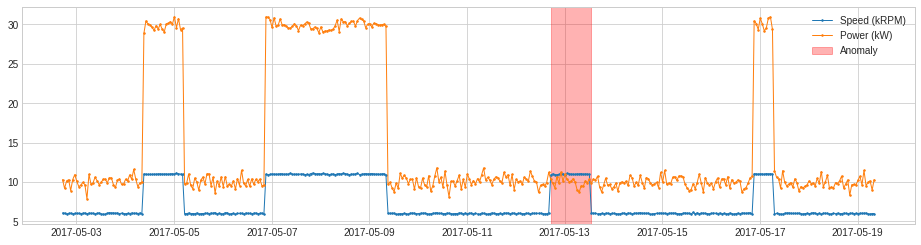### OutlierDetector¶

OutlierDetector performs multivariate time-independent outlier detection and identifies outliers as anomalies. The multivariate outlier detection algorithm could be those in scikit-learn or other packages following same API.

For the same example as above, we apply OutlierDetector with a scikit-learn local outlier factor model.

In :
from adtk.detector import OutlierDetector
from sklearn.neighbors import LocalOutlierFactor
outlier_detector = OutlierDetector(LocalOutlierFactor(contamination=0.05))
anomalies = outlier_detector.fit_detect(df)
plot(df, anomaly=anomalies, ts_linewidth=1, ts_markersize=3, anomaly_color='red', anomaly_alpha=0.3, curve_group='all');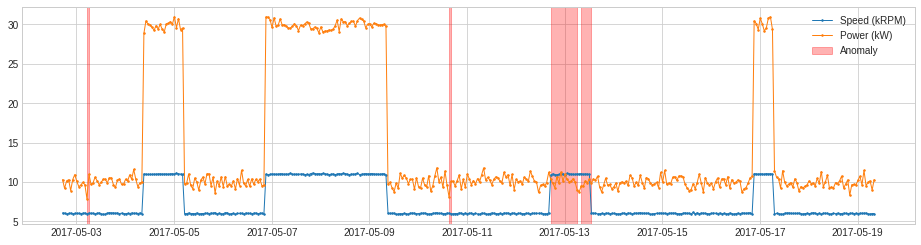### RegressionAD¶

RegressionAD detects anomalous violation of usual relationship between multivariate series by tracking regressive error. Internally, it is implemented as a pipenet with transformer RegressionResidual.

For the same example as above, we apply RegressionAD with linear regression model.

In :
from adtk.detector import RegressionAD
from sklearn.linear_model import LinearRegression
regression_ad = RegressionAD(regressor=LinearRegression(), target="Speed (kRPM)", c=3.0)
anomalies = regression_ad.fit_detect(df)
plot(df, anomaly=anomalies, ts_linewidth=1, ts_markersize=3, anomaly_color='red', anomaly_alpha=0.3, curve_group='all');